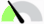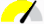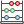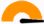# 6.3. Lesson: Network Analysis¶

Calculating the shortest distance between two points is a common GIS task. Tools for this can be found in the Processing Toolbox.

The goal for this lesson: learn to use Network analysis algorithms.

## 6.3.1.Follow Along: The Tools and the Data¶

You can find all the network analysis algorithms in the Processing ► Network Analysis menu. You can see that there are many tools available:

Open the project `exercise_data/network_analysis/network.qgz`. It contains two layers:

• `network_points`

• `network_lines`

The network_lines layer has already a style that helps to understand the road network.

The shortest path tools provide ways to calculate either the shortest or the fastest path between two points of a network, given:

• start and end points selected on the map

• start point selected on the map and end points taken from a point layer

• start points taken from a point layer and end point selected on the map

Let’s start.

## 6.3.2.Calculate the shortest path (point to point)¶

The Network analysis ► Shortest path (point to point) allows you to calculate the shortest distance between two manually selected points on the map.

In this example we will calculate the shortest (not fastest) path between two points.

1. Open the Shortest path (point to point) algorithm

2. Select network_lines for Vector layer representing network

3. Use `Shortest` for Path type to calculate

Use these two points as starting and ending points for the analysis:

4. Click on the button next to Start point (x, y) and choose the location tagged with `Starting Point` in the picture. The coordinates of the clicked point are added.

5. Do the same thing, but choosing the location tagged with `Ending point` for End point (x, y)

6. Click on the Run button:

7. A new line layer is created representing the shortest path between the chosen points. Uncheck the `network_lines` layer to see the result better:

8. Open the attribute table of the output layer. It contains three fields, representing the coordinates of the start and end points and the cost.

We chose `Shortest` as Path type to calculate, so the cost represent the distance, in layer units, between the two locations.

In our case, the shortest distance between the chosen points is around `1000` meters:

Now that you know how to use the tool, feel free to test other locations.

## 6.3.3.Try Yourself Fastest path¶

With the same data of the previous exercise, try to calculate the fastest path between the two points.

How much time do you need to go from the start to the end point?

## 6.3.4.Follow Along: Advanced options¶

Let us explore some more options of the Network Analysis tools. In the previous exercise we calculated the fastest route between two points. As you can imagine, the time depends on the travel speed.

We will use the same layers and starting and ending points of the previous exercises.

1. Open the Shortest path (point to point) algorithm

2. Fill the Input layer, Start point (x, y) and End point (x, y) as we did before

3. Choose `Fastest` as the Path type to calculate

5. Change the Default speed (km/h) from the default `50` value to `4`

6. Click on Run

7. Once the algorithm is finished, close the dialog and open the attribute table of the output layer.

The cost field contains the value according to the speed parameter you have chosen. We can convert the cost field from hours with fractions to the more readable minutes values.

8. Open the field calculator by clicking on theicon and add the new field minutes by multiplying the cost field by 60:

That’s it! Now you know how many minutes it will take to get from one point to the other one.

## 6.3.5.Shortest path with speed limit¶

The Network analysis toolbox has other interesting options. Looking at the following map:

we would like to know the fastest route considering the speed limits of each road (the labels represent the speed limits in km/h). The shortest path without considering speed limits would of course be the purple path. But in that road the speed limit is 20 km/h, while in the green road you can go at 100 km/h!

As we did in the first exercise, we will use the Network analysis ► Shortest path (point to point) and we will manually choose the start and end points.

1. Open the Network analysis ► Shortest path (point to point) algorithm

2. Select network_lines for the Vector layer representing network parameter

3. Choose `Fastest` as the Path type to calculate

4. Click on the button next to the Start point (x, y) and choose the start point.

5. Do the same thing for End point (x, y)

7. Choose the speed field as the Speed Field parameter. With this option the algorithm will take into account the speed limits for each road.

8. Click on the Run button

9. Turn off the `network_lines` layer to better see the result

As you can see the fastest route does not correspond to the shortest one.

## 6.3.6.Service area (from layer)¶

The Network Analysis ► Service area (from layer) algorithm can answer the question: given a point layer, what are all the reachable areas given a distance or a time value?

Not

The Network Analysis ► Service area (from point) is the same algorithm, but it allows you to manually choose the point on the map.

Given a distance of `250` meters we want to know how far we can go on the network from each point of the network_points layer.

1. Uncheck all the layers except `network_points`

2. Open the Network Analysis ► Service area (from layer) algorithm

3. Choose `network_lines` for Vector layer representing network

4. Choose `network_points` for Vector layer with start points

5. Choose `Shortest` in Path type to calculate

6. Enter `250` for the Travel cost parameter

7. Click on Run and close the dialog

The output layer represents the maximum path you can reach from the point features given a distance of 250 meters:

Cool isn’t it?

## 6.3.7. In Conclusion¶

Now you know how to use Network analysis algorithm to solve shortest and fastest path problems.

We are now ready to perform some spatial statistic on vector layer data. Let’s go!

## 6.3.8. What’s Next?¶

Next you’ll see how to run spatial statistics algorithms on vector datasets.